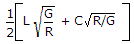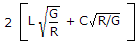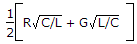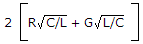# Electronics and Communication Engineering - Electromagnetic Field Theory

1.
Consider an arbitrary distribution of conducting bodies in a charge free space according to the uniqueness theorem, which of following are required to be specified in order that the field is uniquely determined everywhere?
1. Total charge on each conductor
2. Potential at each conductor surface
3. Potential at same of conductor and total charge on the remainder
4. Total charge as well as potential gradient on each conductor surface
Select the correct answer using the codes given below codes
1 and 3
2 and 4
1, 2, and 3
1, 2, 3, 4
Explanation:
No answer description is available. Let's discuss.

2.
The attenuation constant in case of a transmission line isExplanation:
No answer description is available. Let's discuss.

3.
Which of the following is a better propagation mode to propagate television frequency modulation and radar signals?
Satellite communication
Ground propagation
Polarized communication
None of the above
Explanation:
No answer description is available. Let's discuss.

4.
Antenna used for T.V. broadcasting is
long dipole
metal horn
crossed dipole
log periodic